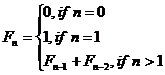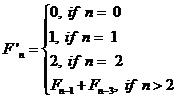Computer Organization and Structure

Homework #2

Due: 2008/10/28

Please write the following three programs in MIPS assembly language.

1.    Triangle Determination (30%):
We will give you three positive numbers as the length for each straight line segment, and your job is to write a program to determine whether these three line segments can form a triangle or not.

Your output should look like this.

----Triangle Determination---

Please type 3 integers, and each with the Enter keys.

N1

N2

N3

Length N1, N2, N3 line segments [CAN / CANNOT] form a triangle.

2.      Variation of Fibonacci sequence (35%):
We all understand the well-known Fibonacci sequence which is defined by the following recurrence relation.…Def. A

Now we make some variations on the original Fibonacci numbers with the new definition as following.…Def. B

In order to let you students get more understanding of how to use assembly language to implement recursive call, you are asked to write a program which computes the modified Fibonacci numbers as Def. B defined.

Your output should look like this

----Variation of Fibonacci ---

Please type 1 integer, and then press Enter keys.

13

The result of F’13 is 101

3.      Prime number finding (35%):
Your third task is to write a program to find the largest prime number which is smaller than or equal to a user-defined number. You should read in a positive number n from the console, then find and display the largest prime number which is also smaller than n. For example, if user input is a positive number 30, and your function should find out the largest prime number within the range [0, 30], which is 23.

Here we introduce the Sieve of Eratosthenes algorithm to solve this problem. The most efficient way to find all of the small primes (say all those less than 10,000,000) is by using the Sieve of Eratosthenes (ca 240 BC):

For example, to find all the primes less than or equal to 30, first list the numbers from 2 to 30.

2 3 4 5 6 7 8 9 10 11 12 13 14 15 16 17 18 19 20 21 22 23 24 25 26 27 28 29 30

The first number 2 is prime, so keep it (we will color it green) and cross out its multiples (we will color them red), so the red numbers are not prime.

2 3 4 5 6 7 8 9 10 11 12 13 14 15 16 17 18 19 20 21 22 23 24 25 26 27 28 29 30

The first number left (still black) is 3, so it is the first odd prime. Keep it and cross out all of its multiples. We know that all multiples less than 9 (i.e. 6) will already have been crossed out, so we can start crossing out at 32=9.

2 3 4 5 6 7 8 9 10 11 12 13 14 15 16 17 18 19 20 21 22 23 24 25 26 27 28 29 30

Now the first number left (still black) is 5, the second odd prime. So keep it also and cross out all of its multiples (all multiples less than 52=25 have already been crossed out, and in fact 25 is the only multiple not yet crossed out).

2 3 4 5 6 7 8 9 10 11 12 13 14 15 16 17 18 19 20 21 22 23 24 25 26 27 28 29 30

The next number left, 7, is larger than the square root of 30, so there are no multiples of 7 to cross off that haven't already been crossed off (14 and 28 by 2, and 21 by 3), and therefore the sieve is complete. Therefore all of the numbers left are primes: {2, 3, 5, 7, 11, 13, 17, 19, 23, 29}. Notice we just found these primes without dividing.

This algorithm can be written in c++ as follows

unsigned int Eratosthenes(unsigned int n) {

unsigned int Sieve;

Sieve = Sieve = 0;

for(unsigned int i = 2 ; i < 500; i++)

Sieve[i] = 1;

for (unsigned int i = 2; i * i < 500; i++)

if (Sieve[i] == 1)

for (unsigned int j = (i + i); j < 500; j += i)

Sieve[j] = 0;

for (unsigned int j = 499; j > 1; j--)

if (Sieve[j] == 1)

return j;

return 0;

}

In the final testing, we will use the number which is smaller than 500 as n.

Your output should look like this.

----Finding Largest Prime Function---

Please type 1 integer which is between 2 and 500, and then press Enter keys.

445

The largest prime between 2 and 445 is 443.